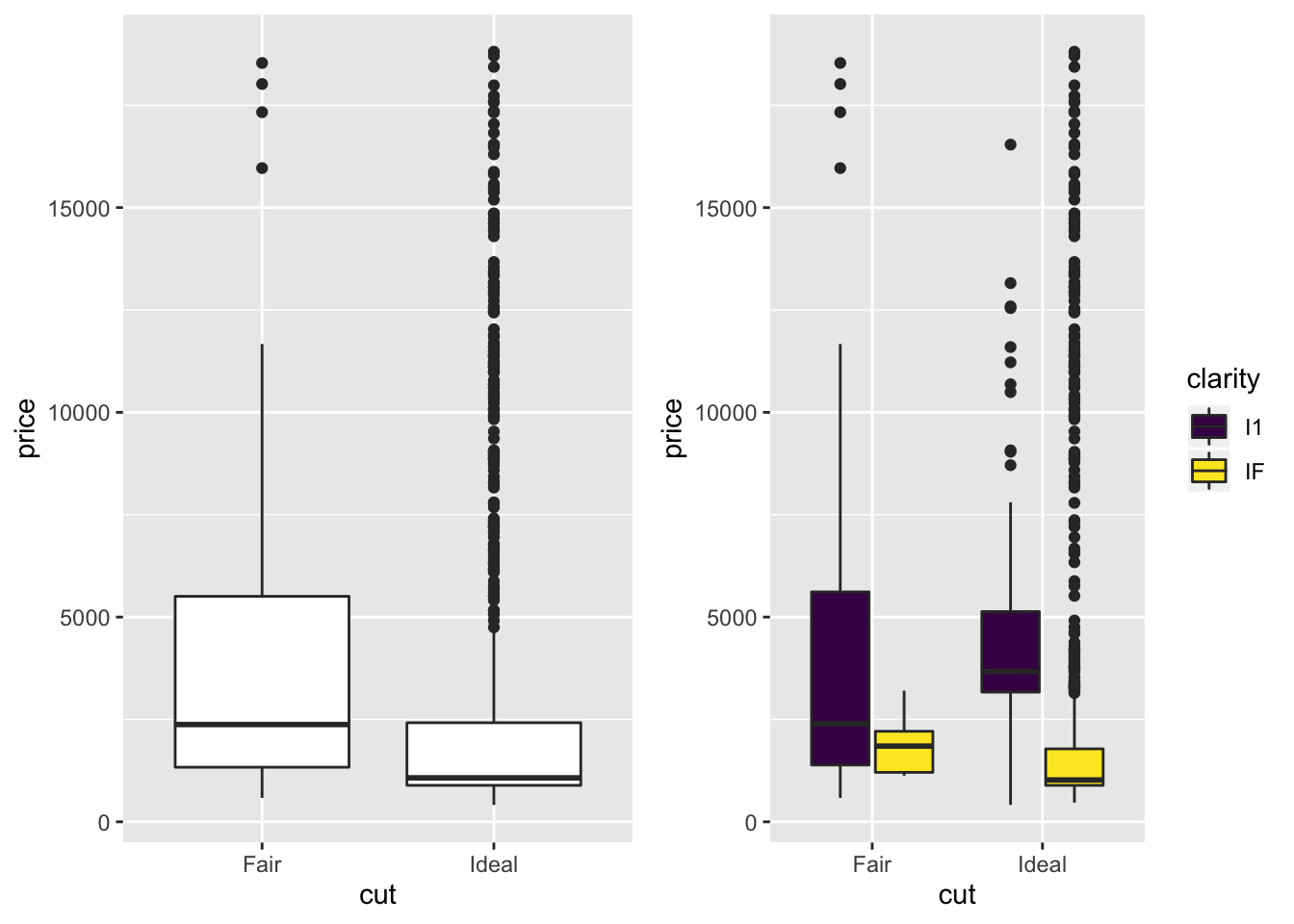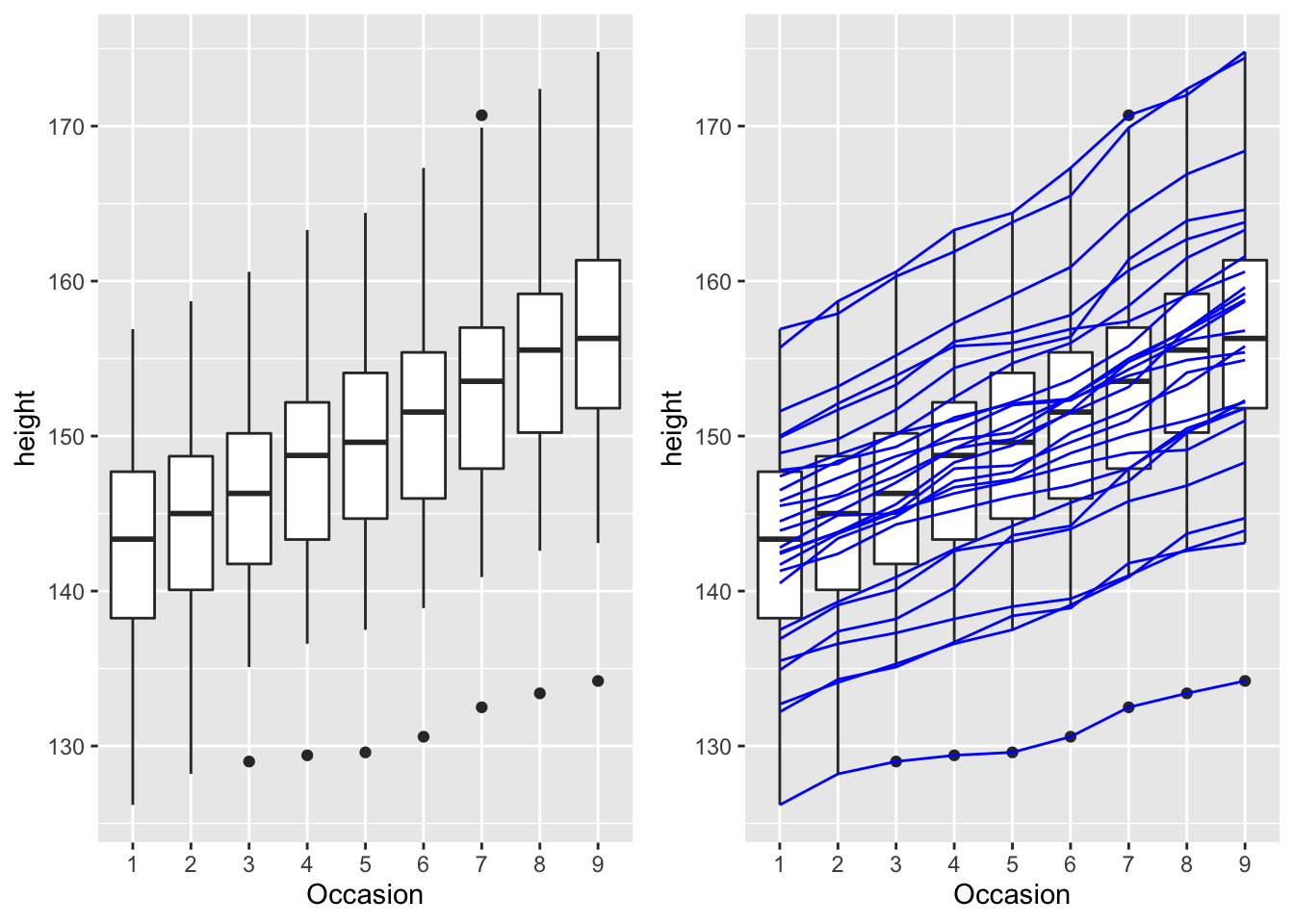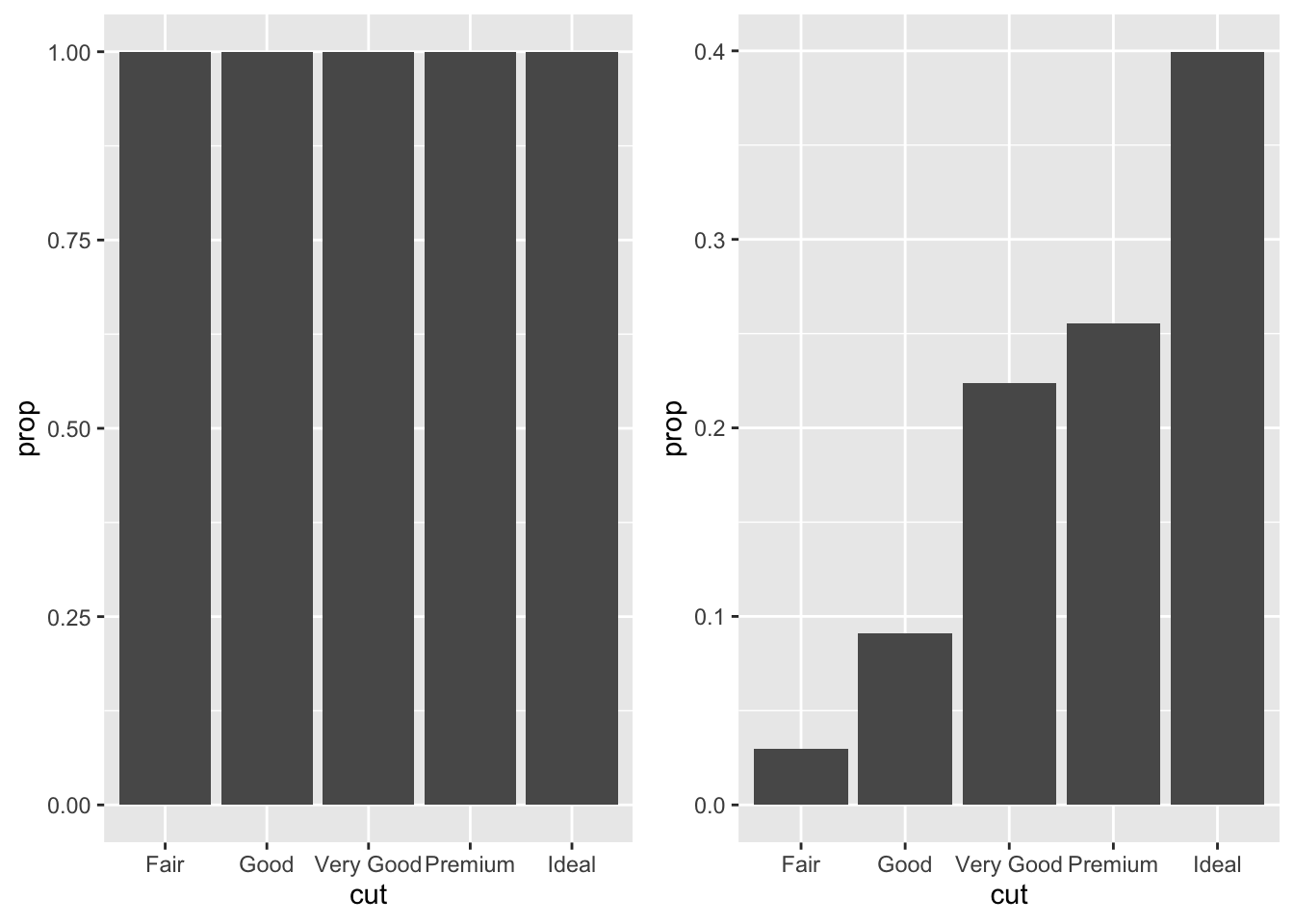# 浅析ggplot aes(group=var)的用法

## 默认分组

• 根据所有离散变量的组合对数据进行分组，如x为离散变量时，根据x的值对data进行分组，等价于dplyr::group(data, x).
• 根据图形属性（映射到分类数据）分组，如fill。以箱型图为例(boxplot)，它用于图形表征离散数据的几个统计特征，默认情况下会根据x轴的分类数据进行分组，如下所示
library(ggplot2)
# sample data
diamonds2 <- dplyr::filter(diamonds,
cut %in% c("Fair", "Ideal"),
clarity %in% c("I1", "IF")
)

p1 <- ggplot(diamonds2, aes(x = cut, price)) +
geom_boxplot()
p2 <- ggplot(diamonds2, aes(x = cut, price, fill = clarity)) +
geom_boxplot()
cowplot::plot_grid(p1, p2, nrow = 1)## 默认分组结果不对，显式指定group

p1 <- ggplot(nlme::Oxboys, aes(Occasion, height)) +
geom_boxplot()
p2 <- p1 + geom_line(aes(group = Subject), colour = "blue")
cowplot::plot_grid(p1, p2, nrow = 1)p1 <- ggplot(data = diamonds) +
geom_bar(mapping = aes(x = cut, y = ..prop..))
p2 <- ggplot(data = diamonds) +
geom_bar(mapping = aes(x = cut, y = ..prop.., group = 1))
cowplot::plot_grid(p1, p2, nrow = 1)